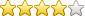Knowledgebase# Why am I seeing negative readings in reports?

SolutionEnergy Data loggers are configured for differential logging. With this, it simply subtracts the current reading from the previous reading, every half hour.

When there is an error reading a meter, then the read is ‘zero’. Thus, for example, current reading (0) minus previous reading (30,000), will result in -30,000. A “negative spike”.

0 – 30,000 = -30000

Also, the negative spike is the meter reading (negative)

Next time around, if there is still an error, you get:

0 – 0 = 0

Eventually when (and if) the meter power/comms are restored, you get a “positive spike” where:

30,000 – 0 = +30000

Likewise, the positive spike is the meter reading.

For more detective work, if the consumption over the period is much less than normal (you’ll have to judge this over past performance), then you can assume a power loss to the meter and/or loads on the meter.

If the consumption is as per the past performance, then the meter is probably powered, but the issue is with Modbus comms.

 Article ID: 78 Category: Problems and Troubleshooting Date added: 2016-02-15 15:00:01 Rating (Votes):(13)